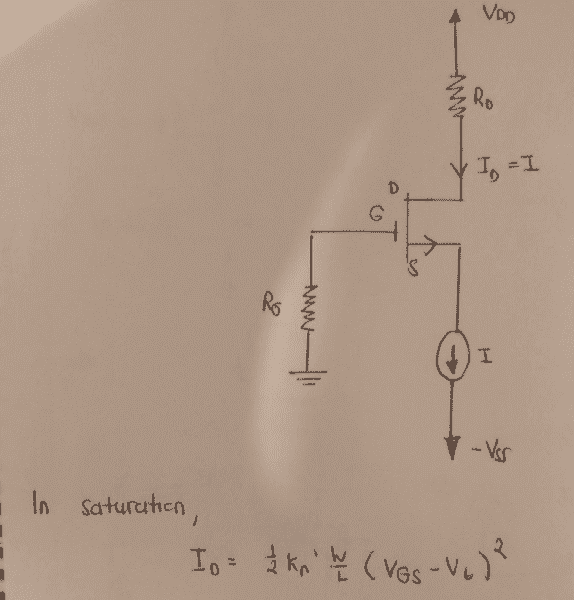# Biasing a MOSFET with constant-current source

## Homework StatementHi,

I'm just going through amplifier circuits and I'm having some trouble with amplifiers in which the MOSFET is biased by a constant current arrangement. Here's my issue:

In the MOSFET saturation equation, if we set ID, we will be able to obtain Vgs. Which means that if we plug in the value of I into that saturation equation, it will tell us what Vgs value we need to have between the gate and source for us to achieve that specified current I as Vgs determines the resistance of the MOSFET internal channel and hence the current.

Now, how come in this arrangement, there is no voltage at the gate? Wouldn't that mean that no channel has been established and hence current could never flow? Or is there assumed to be a DC bias voltage at the gate? If there is a DC bias voltage assumed at the gate, wouldn't it be considered biased by a gate voltage and not by a current source? Or is it assumed that the current source dictates what Vgs bias voltage is required and that whatever bias voltage that current source requires will always be present for it?

I have all these doubts that I can't find answers to.

## Answers and Replies

phyzguy
Science Advisor
You say "there is no voltage at the gate". Sure there is. The gate is grounded, so Vg = 0V. The current source will pull Vs negative until Vgs is sufficiently positive so that the current I flows through the transistor.

You say "there is no voltage at the gate". Sure there is. The gate is grounded, so Vg = 0V. The current source will pull Vs negative until Vgs is sufficiently positive so that the current I flows through the transistor.
So the -Vss at the bottom will cause our Vgs = Vg-Vs to become positive just enough to allow our specified I to flow. How is it possible to dictate how much of that -Vss we get? I mean shouldn't we be getting all of that -Vss at the source then our Vgs would be equal to +Vss and then some really high current could flow?

One more thing, if we replaced that current source with a resistor would that be called a pull-down resistor?

phyzguy
Science Advisor
So the -Vss at the bottom will cause our Vgs = Vg-Vs to become positive just enough to allow our specified I to flow. How is it possible to dictate how much of that -Vss we get? I mean shouldn't we be getting all of that -Vss at the source then our Vgs would be equal to +Vss and then some really high current could flow?

That's what a current source does. It adjusts the voltage until you get the specified current.

One more thing, if we replaced that current source with a resistor would that be called a pull-down resistor?

Yes. In that case, you'd have to calculate how much current would flow.

That's what a current source does. It adjusts the voltage until you get the specified current.

Yes. In that case, you'd have to calculate how much current would flow.

I always thought that an ideal current source produces the specified current no matter what the voltage across it is.

phyzguy
Science Advisor
I always thought that an ideal current source produces the specified current no matter what the voltage across it is.

It does. Note that we are saying the same thing. In the top part of the circuit (the MOSFET and everything) the current depends on Vs. So the ideal current source adjusts Vs until it gets the current I. This changes the voltage across the ideal current source, which is (Vs-Vss). Ideally, the current I is independent of (Vs-Vss), so it is free to adjust Vs to be whatever is needed.

•CoolDude420
It does. Note that we are saying the same thing. In the top part of the circuit (the MOSFET and everything) the current depends on Vs. So the ideal current source adjusts Vs until it gets the current I. This changes the voltage across the ideal current source, which is (Vs-Vss). Ideally, the current I is independent of (Vs-Vss), so it is free to adjust Vs to be whatever is needed.

Ah. I understand now. So in this case, the current source will adjust Vs since it can't change -Vss. And it will adjust Vs to probably some negative value so that Vgs = Vg - Vs = 0 - Vs becomes positive and is greater than the threshold voltage. The exact value of Vs it settles on is dependant on the current I the source is set to.

Would that be correct?

Last edited:
phyzguy
Science Advisor
Ah. I understand now. So in this case, the current source will adjust Vs since it can't change -Vss. And it will adjust Vs to probably some negative value so that Vgs = Vg - Vs = 0 - Vs becomes positive and is greater than the threshold voltage. The exact value of Vs it settles on is dependant on the current I the source is set to.

Would that be correct?

Yes, exactly.

•CoolDude420
Yes, exactly.
That's perfect. Thank you so much!!!